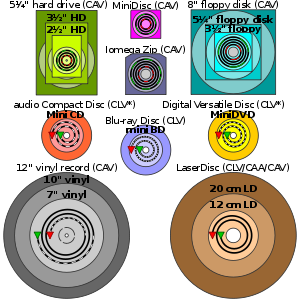# Constant linear velocity

Constant linear velocityComparison of several forms of disk storage showing tracks (not-to-scale); green denotes start and red denotes end.
* Some CD-R(W) and DVD-R(W)/DVD+R(W) recorders operate in ZCLV, CAA or CAV modes.

In optical storage, constant linear velocity (CLV) is a qualifier for the rated speed of an optical disc drive, and may also be applied to the writing speed of recordable discs. CLV implies that the angular velocity (i.e. rpm) varies during an operation, as contrasted with CAV modes. The concept of constant linear velocity was patented in 1886 by phonograph pioneers Chichester Bell and Charles Tainter.

An audio CD employs constant linear velocity to maintain both a constant data rate and a constant bit density. At 1x speed the motor decreases from 495 to 212 rpm as the read head moves away from the center (which is the start of the recording), to keep the disc moving past the read head at a CLV of 1.2 m/s.

To accommodate the higher data transfer rates and random access requirements of modern CD-ROM drives, CAV systems are used. This is because seek performance would be greatly affected during random access by the requirement to continually modulate the disc's rotation speed to be appropriate for the read head's position.

## Zoned Constant Linear VelocityThe Zone-CLV recording strategy is easily visible after burning a DVD-R

Zoned Constant Linear Velocity (ZCLV) is a modification of CLV for high speed CD and DVD recorders. Early model recorders were CLV drives. The recording speed on such drives was rated in multiples of 150 KiB/s; a 4X drive, for instance, would write steadily at around 600 KiB/s. The transfer rate was kept constant by having the spindle motor in the drive vary in speed and run about 2.5 times as fast when recording at the inner rim of the disc as on the outer rim. Some high-speed recorders use the zoned CLV method (ZCLV), which divides the disc into stepped zones, each of which has its own constant linear velocity. At higher speeds, ZCLV offers a compromise between CAV, which enables faster seek times, and CLV, which enables greater storage capacity. A ZCLV recorder rated at "52X", for example, would write at 20X on the innermost zone and then progressively step up to 52X at the outer rim.

## Constant Angular Acceleration

Constant Angular Acceleration (CAA) is a variant of CLV that was used on the Laserdisc format. The initial specification of CLV (as it applied to laserdisc) resulted in several playback artifacts to be present in the audio/video portion as well as compatibility problems with laserdisc players as produced by different manufacturers.

In the mid 1980s, Pioneer Electronics introduced the CAA scheme where the speed in rotation of the laserdisc was lowered in steps and eliminated most playback artifacts and compatibility problems. Since its introduction, most manufacturers of laserdiscs adopted the CAA format but still referred to their CAA-encoded product as CLV.

Wikimedia Foundation. 2010.

### Look at other dictionaries:

• Constant Linear Velocity —   (CLV), CD ROM …   Universal-Lexikon

• constant linear velocity —    Abbreviated CLV. A changing speed of rotation. CDROM disk drives use a CLV encoding scheme to make sure that the data density remains constant. Information on a compact disc is stored in a single, spiral track, divided into many equal length… …   Dictionary of networking

• Constant Linear Velocity — CLV, flow of data at a constant speed (caused by a change in the speed of revolution of the hard disk according to the location of data faster on the inner tracks and slower on the outer tracks) …   English contemporary dictionary

• Constant angular velocity — Comparison of several forms of disk storage showing tracks (not to scale); green denotes start and red denotes end. * Some CD R(W) and DVD R(W)/DVD+R(W) recorders operate in ZCLV, CAA or CAV modes. In optical storage, constant angular velocity… …   Wikipedia

• constant angular velocity —    Abbreviated CAV. An unchanging speed of rotation. Hard disks use a CAV encoding scheme. The constant rate of rotation means that sectors on the disk are at the maximum density along the inside track of the disk. As the read/write heads move… …   Dictionary of networking

• Linear motion — is motion along a straight whiteline, and can therefore be described mathematically using only one spatial dimension. It can be uniform, that is, with constant speed, or non uniform, that is, with a variable speed. The motion of a particle (a… …   Wikipedia

• Linear regression — Example of simple linear regression, which has one independent variable In statistics, linear regression is an approach to modeling the relationship between a scalar variable y and one or more explanatory variables denoted X. The case of one… …   Wikipedia

• Velocity — In physics, velocity is defined as the rate of change of position. It is a vector physical quantity; both speed and direction are required to define it. In the SI (metric) system, it is measured in meters per second: (m/s) or ms 1. The scalar… …   Wikipedia

• Linear elasticity — Continuum mechanics …   Wikipedia

• Constant of motion — In mechanics, a constant of motion is a quantity that is conserved throughout the motion, imposing in effect a constraint on the motion. However, it is a mathematical constraint, the natural consequence of the equations of motion, rather than a… …   Wikipedia